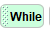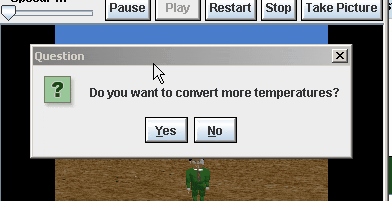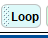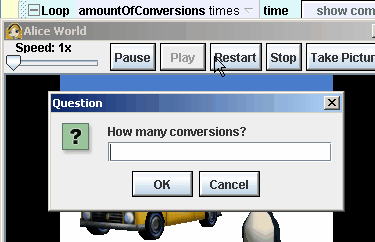# Alice Unit 4 [Loops] Assignments

Assignment 4.1)

Centigrade to Fahrenheit Modification: Modify the Fahrenheit to centigrade project as follows.

1. Use a while loop to do as many temperature conversations as  the user wants2. Ask the user for yes no (help)Assignment 4.2)Miles Per Gallon Modification: Modify the miles per gallon project in the same way as the prior exercise.

1. At the start, ask the user how many mpg conversions,  they want to convert.
2. Then use a loop to do that many conversions4.25 ) Guess Animal’s weight assignment

Assignment 4.3) Open assignment. Your open project must have each of the following criteria

1. a variable
2. store the return value of a function into that variable
3. a loop of some kind

4.4 Rock Paper Scissors